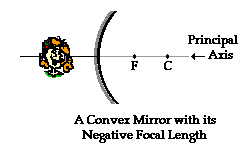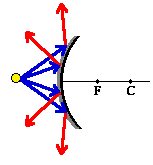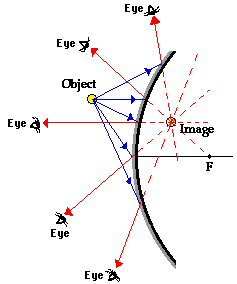Reflection and the Ray Model of Light - Lesson 4 - Convex Mirrors

# Reflection and Image Formation for Convex MirrorsLesson 3 focused on the reflection of light by concave mirrors and on the formation of images by this reflected light. In that lesson, it was shown that concave mirrors can produce both real and virtual images, depending upon the object location. In Lesson 4, we will follow a similar pattern of inquiry for convex mirrors: investigating how convex mirrors reflect light and produce images. We will also investigate how ray diagrams can be used to estimate image location, size, orientation, and type for objects placed in front of convex mirrors. Finally, we will use the mirror equation to calculate numerical information about image distance and size if given an object distance, object size and focal length.

###Anatomy of a Convex Mirror

The diagram at the right depicts a convex mirror. In Lesson 3, a convex mirror was described as a portion of a sphere that had been sliced away. If the outside of the sphere is silvered such that it can reflect light, then the mirror is said to be convex. The center of that original sphere is known as the center of curvature (C) and the line that passes from the mirror's surface through the sphere's center is known as the principal axis. The mirror has a focal point (F) that is located along the principal axis, midway between the mirror's surface and the center of curvature. Note that the center of curvature and the focal point are located on the side of the mirror opposite the object - behind the mirror. Since the focal point is located behind the convex mirror, such a mirror is said to have a negative focal length value.A convex mirror is sometimes referred to as a diverging mirror due to the fact that incident light originating from the same point and will reflect off the mirror surface and diverge. The diagram at the right shows four incident rays originating from a point and incident towards a convex mirror. These four rays will each reflect according to the law of reflection. After reflection, the light rays diverge; subsequently they will never intersect on the object side of the mirror. For this reason, convex mirrors produce virtual images that are located somewhere behind the mirror.

### Reflection and The Formation of Images

Throughout this unit on Reflection and the Ray Model of Light, the definition of an image has been given. An image is the location in space where it appears that light diverges from. Any observer from any position who is sighting along a line at the image location will view the object as a result of reflected light. Each observer sees the image in the same location regardless of the observer's location. As the observer sights along a line, a ray of light is reflecting off the mirror to the observer's eye. Thus, the task of determining the image location of an object is to determine the location where reflected light intersects. The diagram below shows an object placed in front of a convex mirror. Light rays originating at the object location are shown approaching and subsequently reflecting from the mirror surface. Each observer must sight along the line of a reflected ray to view the image of the object. Each ray is extended backwards to a point of intersection - this point of intersection of all extended reflected rays is the image location of the object.The image in the diagram above is a virtual image. Light does not actually pass through the image location. It only appears to observers as though all the reflected light from each part of the object is diverging from this virtual image location. The fact that all the reflected light from the object appears to diverge from this location in space means that any observer would view a replica or reproduction when sighting along a line at this location.

Of course to determine the image location, only a pair of incident and reflected rays need to be drawn. It is customary to select a pair of rays that is easy to draw. Of the five pairs of incident and reflected rays in the diagram above, two correspond to the rays that are customarily drawn. In fact, they may closely resemble the two rays that were used in concave mirror ray diagrams. Recall from Lesson 3 that there were two rules of reflection for concave mirrors. They are:

• Any incident ray traveling parallel to the principal axis on the way to a concave mirror will pass through the focal point upon reflection.
• Any incident ray passing through the focal point on the way to a concave mirror will travel parallel to the principal axis upon reflection.

The revised rules can be stated as follows:

• Any incident ray traveling parallel to the principal axis on the way to a convex mirror will reflect in such a manner that its extension will pass through the focal point.
• Any incident ray traveling towards a convex mirror such that its extension passes through the focal point will reflect and travel parallel to the principal axis.

In the diagram above, the second and third (from the top) blue incident rays exemplify these two rules of reflection for convex mirrors. Using this pair of incident and reflected rays will greatly simplify the task of drawing ray diagrams and determining the location of images. In the next section of this Lesson, such ray diagrams will be shown.

### We Would Like to Suggest ...Why just read about it and when you could be interacting with it? Interact - that's exactly what you do when you use one of The Physics Classroom's Interactives. We would like to suggest that you combine the reading of this page with the use of our Optics Bench Interactive or our Name That Image Interactive. You can find this in the Physics Interactives section of our website. The Optics Bench Interactive provides the learner an interactive enivronment for exploring the formation of images by lenses and mirrors. The Name That Image Interactive provides learners with an intensive mental workout in recognizing the image characteristics for any given object location in front of a curved mirror.

Next Section: